# Neighbor spacing of zeros of L-functions

We use the notation from Distribution of zeros of L-functions, and everything below assumes RH and is specialized to the case of the Riemann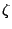-function.

Write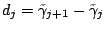for the normalized difference between consecutive zeros of the-function. The GUE conjectures imply that for all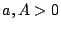we have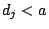for a positive proportion of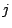, and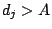for a positive proportion of. There have been a number of efforts aimed at showing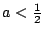because this would prove the nonexistence of Siegel zeros. (See [49 #2590] for a reference). At present the best results, which are due to Soundararajan [ MR 97i:11097], are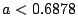and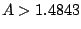.

The GUE conjectures also imply that for all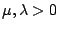we have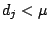for infinitely many, and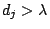for infinitely many. At present the best results (which assume RH and GLH) are [ MR 88g:11057]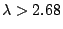and [ MR 86i:11048]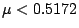. Unconditionally, Richard Hall (unpublished) has shown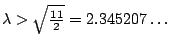.

It has not been shown that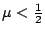implies the nonexistence of Siegel zeros. However, Conrey and Iwaniec have recently shown that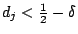, for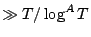zeros with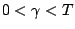, implies the nonexistence of Siegel zeros.

As described in the article on The Alternative Hypothesis, the possibility of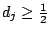, for all, is consistent with everything which is known about the correlation functions of the zeros of the-function. However, it is possible that there is a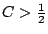such that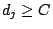, for all, is also consistent with current information on the correlation functions. It would be interesting to know the correct answer.

Back to the main index for L-functions and Random Matrix Theory.